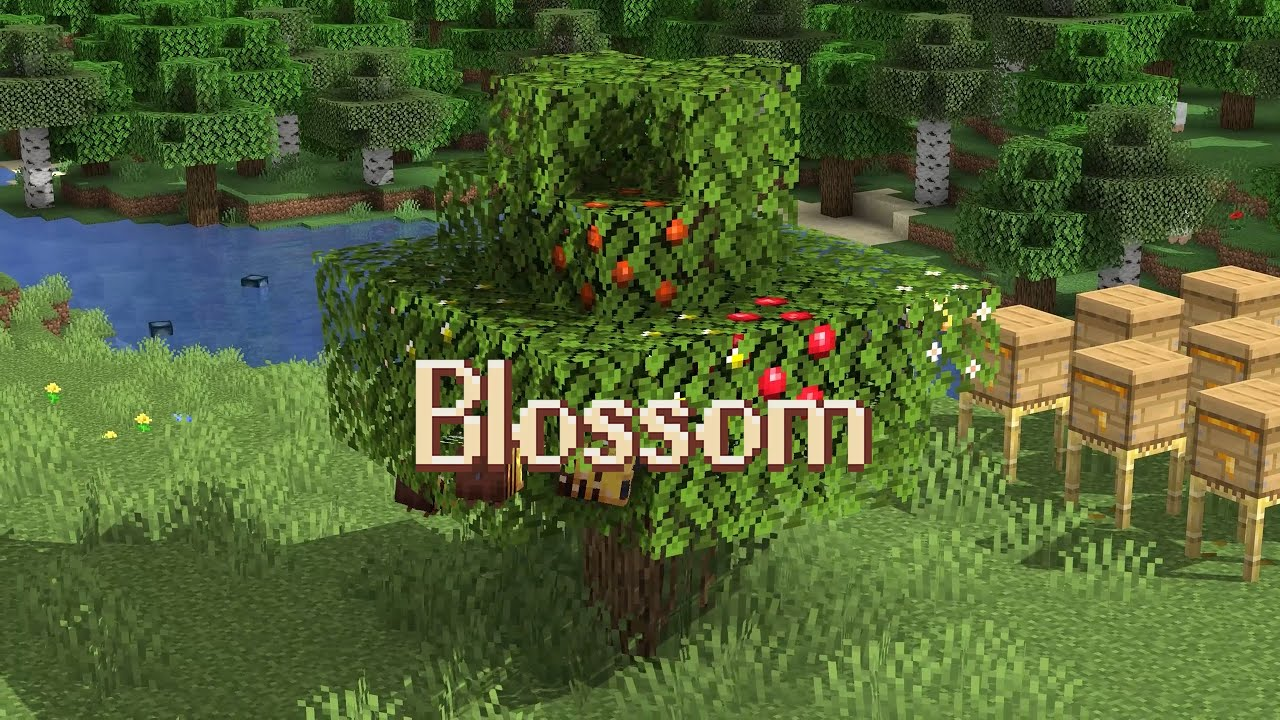# Blossom

Fruit blossoms from trees!

FoodUtility

Created 6 months ago
Updated 2 months ago

### Technical information

Client side
required
Server side
required
Project ID
iYVih5quBlossom is a Fabric mod for Minecraft that makes oak leaves blossom and bear fruit! No longer do you have to destroy your tree to get those apples, now you can simply shear them off when they are grown! Flowering oak leaves will bloom from any oak leaves when pollinated by bees. Watch as bees nurture your apples from bud to pome! You can help speed up their growth with bone meal, if you so choose.

− × − × − × − × − × − × − × − × − × − × − × − × − × − × − × − × − × − × − × − × − × − × − × − × − × − × − × −

What does this do?

It adds a Flowering Oak Leaves block that Oak Leaves can turn into. These flowering leaves will grow until they bear fruit, which you can then shear to obtain! Upon shearing, these flowering leaves will return to normal leaves until they start the process over again!

Why do you need this?

If you want to preserve your trees and still get apples, or if you are seeking more realistic apple harvesting!

What does it require?

− × − × − × − × − × − × − × − × − × − × − × − × − × − × − × − × − × − × − × − × − × − × − × − × − × − × − × −